PlantEye F500 measures the reflectance of each point in 4 different wavelengths. Each of those wavelengths is measured in one channel. The standard wavelengths and their according channels are:

Channel                     Peak Wavelength     Spectral Half Width
Red (R)                       620 - 645 nm            20nm
Green (G)                   530 - 540nm             30nm
Blue (B)                      460- 485nm              20nm
Near-Infrared (NIR)    820- 850nm             30nm

# Spectral Indices

Based on the above mentioned channels different indices are computed directly after the measurement and are based on specific plant wavelength absorption features.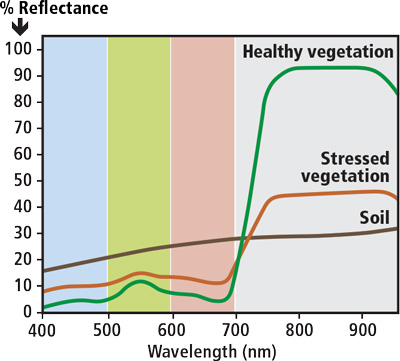### NDVI

Value Range: -1–  1

• Normalized differential vegetation index
• (NIR – RED)/(NIR + RED)
• Correlated to PAR interception

NDVI is computed as the ratio of the NIR and the Red channel in PlantEye. It is computed as the difference of both channels divided by the sum of both channels. Therefore it is a ratio in the range of -1 to 1. The illustration below shows three different examples for the calculation of NDVI. The X axis represents a simplified spectrum in the range from 400 to 1,000nm. The Y axis shows the relative reflectance for each wavelength. The colored blocks represent the measurement channels of PlantEye with standard configuration.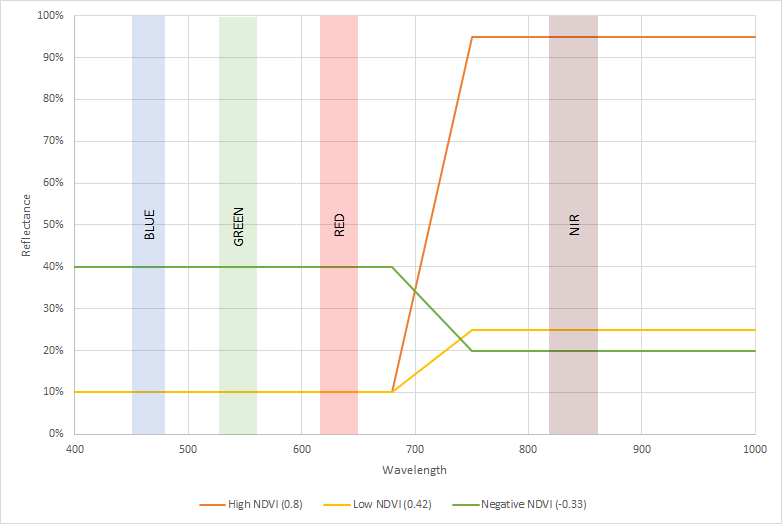One can see that there are three different examples that show the value for the NDVI index. The first example shows a high NDVI where the reflectance in the NIR channel is much higher than in the Red channel. This is the typical effect for healthy vegetation. The index is around 0.8. The second example shows a low NDVI. In this case the reflectance in the NIR channel is only slightly bigger than in the Red channel. This is a typical pattern for unhealthy vegetation. The last example shows that in theory the NDVI value could also be negative if the Red reflectance is higher than the NIR reflectance. This is typically not observed in any plant related measurements.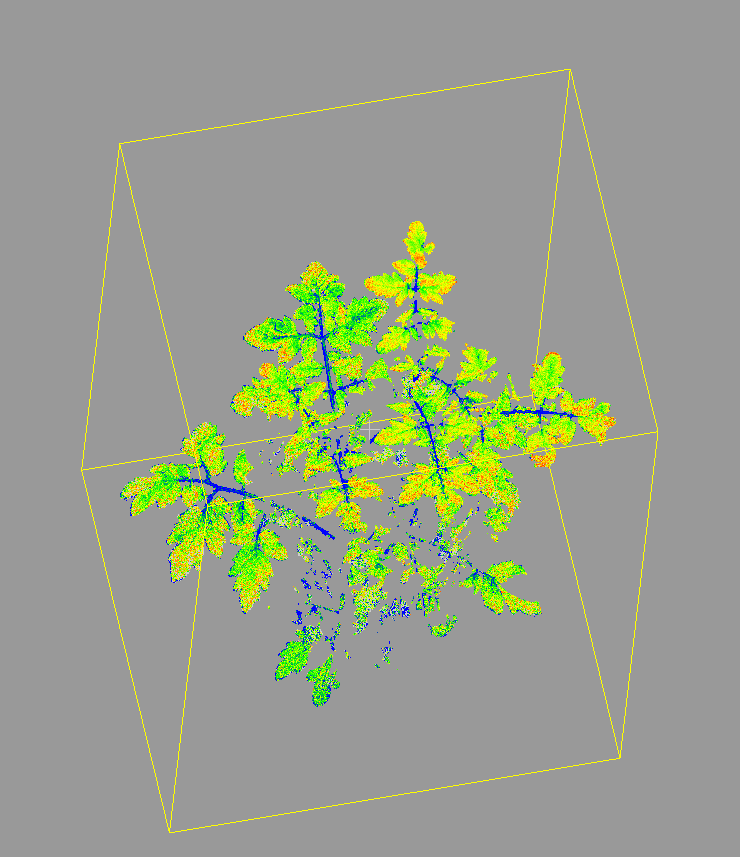### NPCI

Value Range: -1–  1

• normalized pigment chlorophyll ratio index
• (RED − BLUE)/(RED + BLUE)
• Correlated to chlorophyll content

### PSRI

Value Range: -1–  1

• plant senescence reflectance index
• (RED − GREEN)/(NIR)
• Correlated to Carotenoid/Chlorophyll ratio

### Greenness

Value Range: -1–  1

The greenness index represents the relation between the reflectance in the green channel compared to the other two visible light channels (red and blue). It is computed as (2*G-R-B)/(2*G+R+B). The higher the green reflection compared to the other channels the higher the greenness index. A value of -1 would represents a full reflection in the green channel and no reflection in the other two channels. The illustration below shows three different examples for the calculation of greenness. The X axis represents a simplified spectrum in the range from 400 to 1,000nm. The Y axis shows the relative reflectance for each wavelength. The colored blocks represent the measurement channels of PlantEye with standard configuration.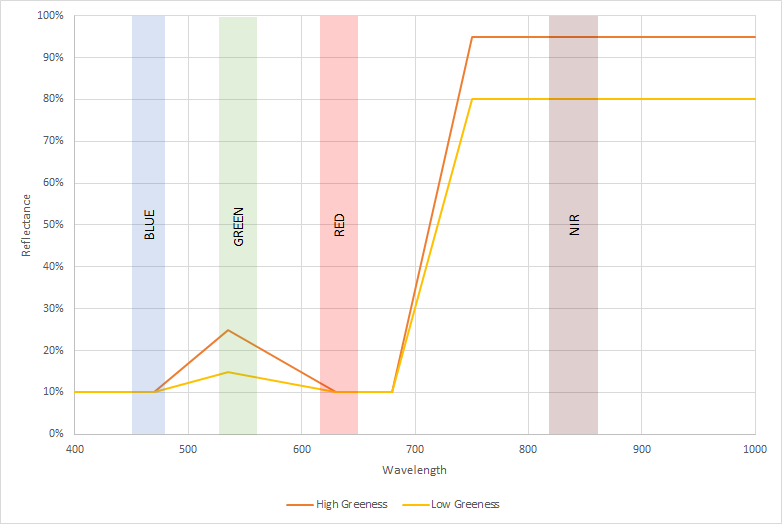### Hue

Value Range 0 - 360

The standard approach in technical devices is to store colors as a combination of red, green and blue values. Nevertheless, this color model does not well represent the color of object. Each individual color is a combination of red, green and blue values.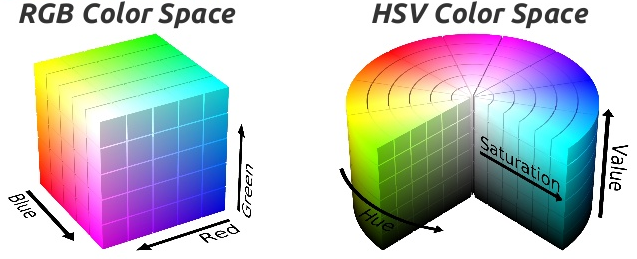As an alternative approach to RGB color spaces the HSV color space was developed. In this color space the idea is to express the color (hue) on one dedicated channel that is arranged in the rainbow colors. The saturation of each color represents if it is a pale or very intense color. Last, the Value shows if the color is dark or bright. The advantage of this color space is that a color can be expressed independently from its saturation or value. The example below shows an image of what plots decomposed into the red, green and blue channel as well as decomposed into hue, saturation and value. One can see that it is hard to see the difference of the plot color in the red, green and blue channels whereas the hue channel nicely identifies the color differences of the plots on the left hand side of the image.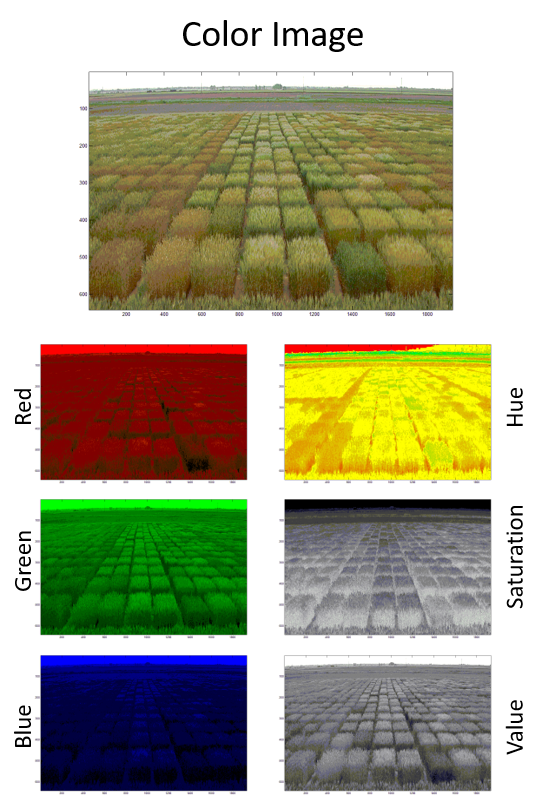PlantEye can compute the hue channel for the point cloud and analyze it further. Therefore, the hue channel can be seen as a color analysis taking into account all three measured colors.

# Index Analysis

Each of the above mentioned indices is analyzed in PlantEye F500 on two different ways. The first way is a simple average computation of the index over the entire sector (e.g. the plant or the plot). In the average approach all points that belong to a certain sector are averaged to represent a general overview of the sector.

Another approach is to look not only at the average value but also to look at the distribution and further divide it into regions of interest - so called bins.  The idea of a bin is to count how many points of the sector are within the boundary of a bin. Each bin therefore has a lower and an upper limit. With these limits we count the number of points within and express them as a percentage of the total area.

The illustration below further illustrates this approach based on a binning for the hue index. The image shows the distribution of the hue values for the two trays of wheat shown on the right hand side. The X axis represents the hue values from 0 to 360. The y axis shows what proportion of the plant has this specific hue value. The same approach can be done on the other indices such as NDVI or Greenness.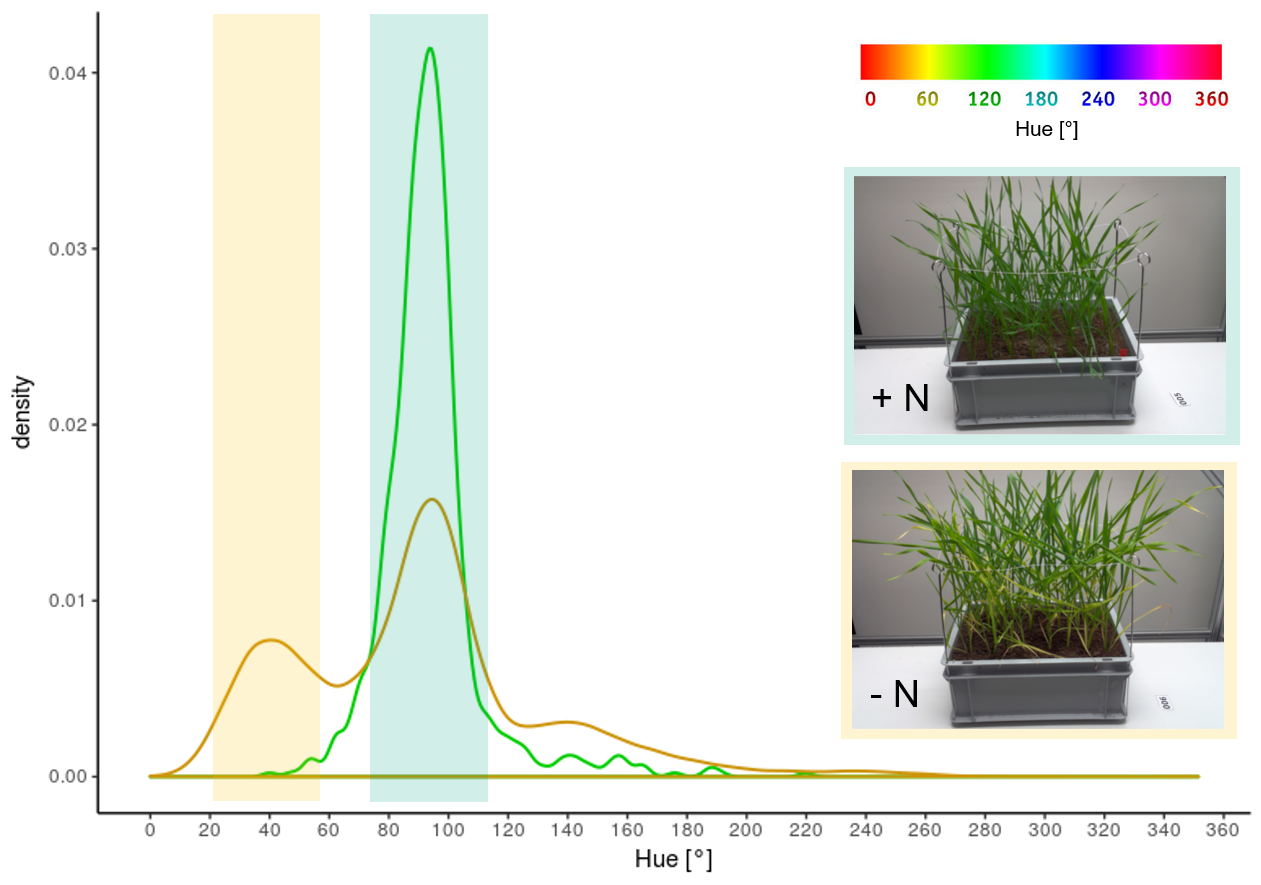One can see that the healthy plants (green line) have their main peak between 80 and 110 whereas the nitrogen deficit plants have an additional peak between 20 and 60. The idea of the binning is now to simplify this distribution of the hue channel into clusters of color. To do that we have defined the yellow and green cluster visualized by the boxes in the histogram with the upper and lower threshold set to the above mentioned values. The result of the binning for this cluster now will be the percentage of points that fall into these bins. For the healthy plants we would have 90% of their total area in the green bin and less than 2% of their area in the yellow bin. The nitrogen deficit plant will have only 40% in the green bin and 20% in the yellow bin. The remaining percentage is outside the bins and ignored for further analysis.

The bins can be defined arbitrarily. Up to 5 bins can be defined for each channel. The value for each bin is the percentage of the total 3D leaf area. If you want to recompute the absolute area that falls into this bin simply multiply the percentage area with the 3D leaf area to transform back into mm².